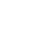Live chatQ1.  Prepare a NPV table based on the information given and using the table BFBS Cost Analysis format

Given:

New Machine Cost = \$40,000

Working Capital = \$3,000

Project Life = 10 years

Annual Net Cash Flow = \$15,000 each year (Ordinary Annuity)

Discount Rate = 10 %

Tax Rate = 40 %

Salvage Value = \$6,000

Depreciation = Straight line over 10 years.

## Yearly depreciation calculation

Cost = \$ 40,000

Salvage value = \$6,000

Cost – Salvage value = \$40,000 - \$6,000 = \$34,000

10 – year straight line depreciation = \$34,000 / 10 = \$3,400 per year.

Assumptions:

1. Annual net cash flow = Sales revenue – (Cost of goods sold + Salaries, Shipment, etc.).
2. Cash inflows are even
3. Depreciation value is not added to expenses
4. Earning before income taxes (EBIT) is calculated without depreciation
5. Effect of depreciation is added to the NPV calculation as an additional earning, and is shown in the BFBS cost analysis table
 BFBS Cost Analysis Years Amount Tax Effect 40% After-Tax Cash Flows 10% Factor Net Present Value Cost of asset Now (\$40,000) (\$40,000) 1.0000 (\$40,000) Working capital needed Now (\$3,000) (\$3,000) 1.0000 (\$3,000) Net annual cash inflows 1 to 10 \$15,000 0.6 \$9,000 6.14457 \$55,301 Depreciation tax shield 1 to 10 \$3,400 0.4 \$1,360 6.14457 \$8,357 Salvage value 10 \$6,000 0.6 \$3,600 0.38554 \$1,388 Working capital released 10 3000 3000 0.38554 \$1,157 Net present value \$23,202

## Q2.  Analyze the data in the NPV tableNet Present Value (NPV) calculates the present value of an investment using the discounted sum of all cash in flows and out flows to and from the project.  It also considers salvage value and released working capital, if any.  The principal parts of NPV are investment, cost of the capital or discount rate, and cash inflows throughout the project life (Managerial Accounting).

To initiate the project, BFBS needs to invest \$40,000 to purchase a new machine, and \$3,000 working capital to maintain the machine.  These are cash out flows.  In the NPV calculation, they are negative.

The project life is 10 years.  Throughout the project life, it generates cash from the operation.  In this case, it generates \$15,000 each year.  However, out of \$15,000, the company pays 40 % taxes.  Thus, 60 % goes to the company as cash inflow.  In NPV this value, it is positive.

Mentioned above assumptions stated that depreciation was not added to the expenses in net cash flow evaluation.  Depreciation through the tax shield affects taxes, and it indirectly affect after tax cash flows (Wiley).  Depreciation, in this case, gives 40 % tax shield, which is a cash inflow.  In the NPV calculation, in this case, is a positive value.

Limited time Offer

0
0
days
:
0
0
hours
:
0
0
minutes
:
0
0
seconds
Get 19% OFF

NPV considers time value factor of the future cash flows.  Net Present Value (NPV) is calculated based on the following formula (Net Present Value).

NPV = - C0 + {C1/(1+r)} + {C2/(1+r)2} + {C3 / (1+ r)3} + ……  +{Ct / (1+r)t}; where,

C0 = Initial investment;

C1 = Net annual cash flow for year 1;

C2 = Net annual cash flow for year 2;

C3 = Net annual cash flow for year 3,

Ct = Net annual cash flow for year t.

r = Discount rate

t = Time

In the above formula, 1 / (1+r)t considers the time value factor of money.  The ratio, 1 / (1+r)t is called PV factor.  It depends on “r”, the discount rate.  PV factors for 10 years with the discount rate of 10 % are shown below in the tabular form.

 10.00% PV Factors Year 1 0.90909 Year 2 0.82645 Year 3 0.75131 Year 4 0.68301 Year 5 0.62092 Year 6 0.56447 Year 7 0.51316 Year 8 0.46651 Year 9 0.42410 Year 10 0.38554

The present value of the future money is calculated by multiplying it with PV factors.  The sum of all PV factors at 10 % discount rate for 10 years is 6.14457.  Net cash inflow and depreciation used this factor to evaluate the present value of the future moneys.

Stay ConnectedSalvage value is an income for the company.  The company pays 40 % tax of this income and generates 60 % cash inflow.  In NPV calculation, it is a positive value.  Its present value is calculated by multiplying PV factor of the 10th year, which is 0.38554.

Released working capital is not an income.  That is why; the company does not pay taxes.  On the other hand, its value after 10 years is not same as of its current value.  That is why; released working capital is multiplied by PV factor of the 10th year, which is 0.38554.

For the BFBS project, NVP is the sum of all cash flows shown in Table 1.  The NPV of the project is \$23,202.

Q3.  Discuss the decision that should be made concerning the investment in the new machine.

NPV is a capital budgeting evaluation method.  Industry recommendation is to accept the project if the NPV value is positive (Managerial Accounting).  In this case, the NPV value is \$23,202.  The BFBS Company can accept the project.

0

Preparing Orders

0

Active Writers

0%

Positive Feedback

0

Support Agents

Limited offerget 15% off your 1st order with code first15